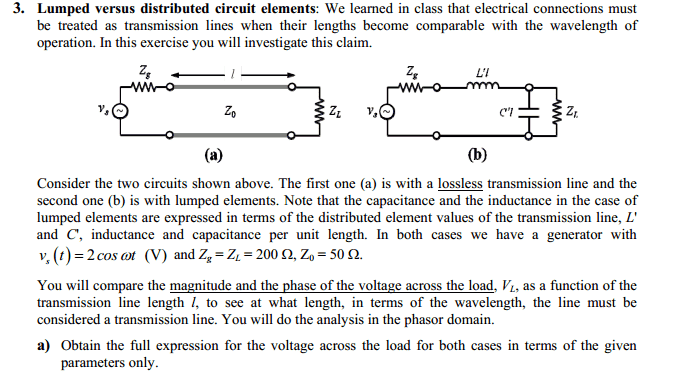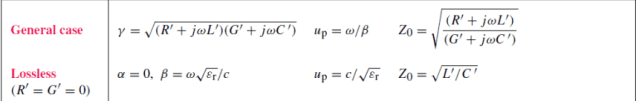# Find V(L) for Lumped vs distributive circuits

• Engineering
nagr

## Homework Statement## Homework Equations

Vg=2cos(wt)
Zg=Zl=200
Zo=50
$\Gamma$=$\frac{Zl-Zo}{Zl+Zo}$
L'R'=με (all TEM lines)
μ0=4∏E-7
ε0=8.854E-12## The Attempt at a Solution

Basically I think that with the 1st one, i don't have to deal with reflection or whatever. i could be wrong, since Zo does not equal Zl in both problems, which means there has to be reflection. but the equation I used for the 1st problem was in my book, and its similar to the voltage source equation. 2cos(wt-θ) where θ=wl/c. Since we have to graph V(L) for both as a function of length which is in terms of wavelength, this is also listed in book as θ=2∏*(l/λ). The only other thing that confuses me is that frequency is not given, so i can't find w. How can I vary both length (in terms of wavelength) and frequency, which is nowhere mentioned in the problem?? maybe i am doing this wrong.

for the 2nd problem. i think this is basically the same as the 1st one, except now we deal with reflection. since we also deal with R' L' G' C' (with R'=G'=0). the main breakthrough ive had with this problem is finding $\Gamma$=0.6 which was easy. But i have no idea what to do from here. if I ASSUME that the relative permittivity is the same as in in air (that is εr=1) THEN i calculate R' L' using the equations Zo=50=sqrt(L'/C') and β=w*sqrt(L'C')=w*sqrt(με), and if i do that I get C'=6.671E-11 and L'=1.668E-7 but, i dont want to assume anything. can someone help me? i have the equations but theres no problem like this in my book, plus im lacking values like frequency and εr, so im not sure what exactly to do.

Last edited:

Homework Helper
Gold Member
1st problem: there is definitely reflection, not that that's important here.
What equation relates load voltage to source voltage with the given parameters w, L', C', l, RL, RG, Z0?

2nd problem: this is a lumped circuit. There are by definition no reflections with lumped circuits.

nagr
the only equation i see in my book is V(z)=V0+(e-jβz)+V0-(ejβz)
at the load voltage VL is at z=0 and given by V0+ + V0-
V0+/V0- are related to reflection coefficient by: V0- = $\Gamma$V0+

to find V0+ i would have to find Zin. where Zin=Z0[(zL+jtanβl)/(1+j(zLtanβl)] where zL=ZL/Z0=4 and β=w*sqrt(L'C')=w*sqrt(με). So I have Zin=50[(4+jtanβl)/(1+j(4tanβl)] but i have trouble finding β without L' and C'. my book also has another equation β=2∏/λ but im not sure if i should use that.

Last edited:
Homework Helper
Gold Member
Your answer will include ω, L' and C', also l plus the given parameters Zg, ZL. You can't get numerical answers without those but you can compare V(0) for a transmission line vs. the lumped circuit.

You also have the relations in that post, plus β = ω√(L'C') and Z0 = √(L'/C').